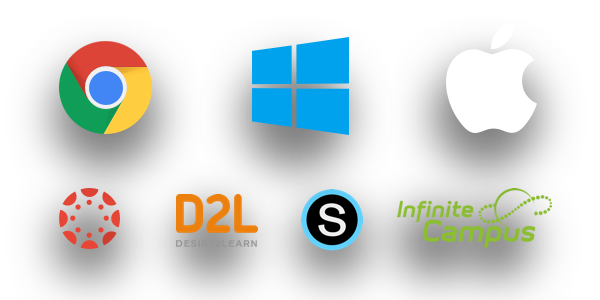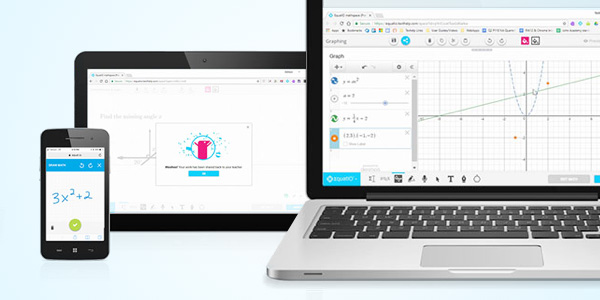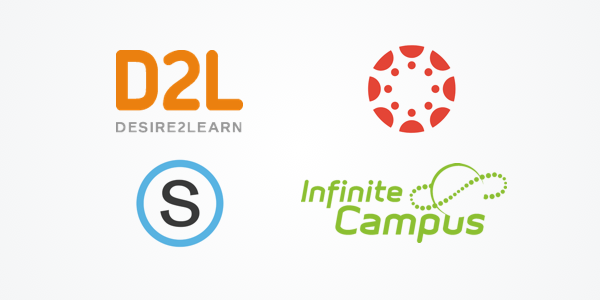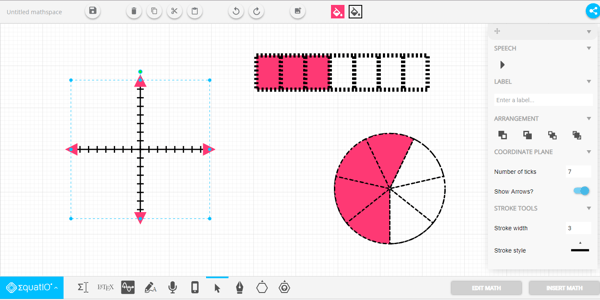TextHelp Equatio

Editmicro » TextHelp EquatioFREEGDS

FREEGDS

Make math digital

EquatIO allows you to create equations, formulas, and more, digitally. Helping to make math and STEM classes more accessible and engaging for every student.

EquatIO is free for teachers!

We offer our digital math tool free for K-12 teachers. This means you can get a premium subscription of EquatIO for free!

Use EquatIO to

EquatIO gives your students the opportunity to show their mastery in math, in a way that best suits them. They can speak, draw or type problems and equations directly onto their device.

Make math accessible

EquatIO brings math out of the pen and paper era. By moving to digital instruction, EquatIO makes math more accessible. Students with learning difficulties or visual impairments can hear their math read aloud to them.

Help visualize math problems

Students and educators can create and customize graphs with EquatIO. Powered by Desmos graphing calculator, EquatIO helps students to visualize and explore a written equation.

Features – at a glanceEquation editorCreate your math and science expressions by typing right into the editor. Use Prediction to insert fractions, exponents, operators, formulas, chemical symbols, and more.Speech input: Dictate equations and formulas aloud. EquatIO understands what you’re saying, turning your spoken input to written expressions.Graph Editor: Create and customize single or multiple graphs, plot ordered pairs or tables of points and more, with this tool powered by Desmos graphing calculator.Screenshot reader: Turn any equation across the web into accessible, editable math with the EquatIO Screenshot Reader.Handwriting recognition: Handwrite math expressions using a touchscreen device or mouse pointer.

A helping hand where it’s needed

EquatIO works across lots of different platforms including Google, Windows, and Mac. It also integrates seamlessly with many popular learning management systems. This means that your students can work with EquatIO in familiar environments.Making math digital

EquatIO has the power to make math digital and inclusive for all your students. With the ability to handwrite, type or dictate math problems directly into a device, it no longer needs to be a pen and paper lesson.Integrate with popular LMS & classroom software

Moving to digital math needn’t be daunting. With EquatIO students can continue to use all the programs they’re familiar with as EquatIO has many seamless integrations. Teachers can continue to use the Learning Management systems they’re used to as well, such as Schoology, Brightspace D2L, Canvas and Infinite Campus.Create collaborative maths resources for teachers & students

Turn problem solving into problem sharing! EquatIO® mathspace is a collaborative workspace that enables you and your students to make maths together. It helps you to bring mathematics to life for the whole class.